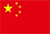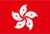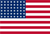Scanning QR Code• Online Inquiry

+
Congratulations
You've successfully got advanced quotes worth 5000 HKD
• Home
• Quotes
• News
• Community
• Learn
• Help Center
• Language
•••EN

# Basic concepts of a stock

## Basic field

### 1. Market value

Formula: Market value = total equity × latest price

Meaning: refers to the total stock value of a listed company's total shares calculated at market prices

### 2. Current value

Formula: Current value = tradable shares × latest price

Meaning: refers to the stock value of a listed company's shares circulating in the market calculated at market prices

### 3. Total equity

Meaning: the total equity is the total number of shares issued by the stock company.

Meaning: tradable equity refers to the shares of a listed company that only circulate in the market.

Note: For ADS-listed stocks, the outstanding shares are calculated and no official information is available.

### 5. Turnover rate

Formula: Turnover rate = (number of shares traded / number of shares outstanding at the time) × 100%

Meaning: Refers to the frequency of stocks changing hands in the market within a certain period of time, and is one of the indicators that reflect the strength of stock liquidity.

### 6. Amplitude

Formula: Amplitude = (the price of the highest point of the day - the price of the lowest point of the day) / the closing priceyesterday× 100%

Meaning: the absolute value of the difference between the highest and lowest price of the day after the opening of the stock and the percentage of the previous day's closing price, which shows the activity of the stock.

### 7. Committee

Formula: Committee= (commission to buy the number of lots - commission to sell the number of lots) / (commission to buy the number of lots + commission to sell the number of lots) × 100%.

Meaning: The ratio of all orders on the quotation system, used to measure the relative strength of orders over a period of time.

### 8. Volume ratio

Formula: Volume Ratio = (Current total lots traded / Current cumulative opening time (minutes)) / Average volume per minute over the past 5 days.

Meaning: The ratio of the average volume per minute since the market opened to the average volume per minute over the past 5 trading days. Volume ratio is a measure of relative volume.

## Financial field

### 1. P/Eratio (static)

Formula:

"Hong Kong stocks, A shares" P/E ratio (static) = total market value for the day / profit attributable to shareholders of the previous fiscal year

"US stocks" P/E ratio (static) = latest price/diluted earnings per share disclosed in the latest annual report

Meaning: refers to the ratio of the market price divided by the earnings per share disclosed in the latest annual report.

The static price-earnings ratio ignores short-term changes and is calculated using the financial data of the latest annual report, so this indicator lags behind the immediate situation of the company.

### 2. P/E ratio TTM

Formula:

"Hong Kong stocks, A shares" P/Eratio (TTM) = total market value on the day / profit attributable to shareholders in the past 12 months

"U.S. stocks" P/E ratio (TTM)= latest price/diluted EPS disclosed in last 12 months statement

Meaning: refers to the ratio of the market price divided by the earnings per share disclosed in the most recent 12-month statement. The earnings per share in the rolling price-earnings ratio uses the financial data of the past four quarters. Although its starting point will change, and these four quarters may belong to two different natural years, it still makes up for the objective seasonality of listed companies.

The impact of the difference makes up for the timeliness of the static P/E ratio using year-on-year data.

### 3. P/Bratio

Formula:

P/Bratio = latest price/net assets per share attributable to ordinary shareholders

Meaning: Refers to the net assets attributable to ordinary shareholders per share based on the market price ratio.

The generalized net assets are not selected for calculation to reflect the corresponding equity of ordinary shares. Among them, the net assets per share is the ratio of common shareholders’ equity in the latest reported balance sheet to the total share capital.

### 4. Dividend yield

Formula:

Dividend(TTM)= total dividends of the last 12 months / total share capital of the day

Dividend (LFY)= total dividends of the previous fiscal year / total share capital of the day

Dividend yield (TTM)= total dividends in the past 12 months / total market value on the day

Dividend yield (LFY)= total dividends of the previous fiscal year / total market value of the day

Implication: the proportion of net income attributable to dividends, which reflects the company's dividend distribution policy and dividend-paying ability.

Back to the Top